• Get access

Do you like the article?
Share it with others -

Use new possibilities of MetaTrader 5

# MQL4 Language for Newbies. Introduction

21 June 2007, 15:14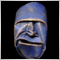12
20 309

### Introduction

This sequence of articles is intended for traders, who know nothing about programming, but have a desire to learn MQL4 language as quick as possible with minimal time and effort inputs. If you are afraid of such phrases as "object orientation" or "three dimensional arrays", this article is what you need. The lessons are designed for the maximally quick result. Moreover, the information is delivered in a comprehensible manner. We shall not go too deep into the theory, but you will gain the practical benefit already from the first lesson.

If you have never worked with programming before, you may find it difficult to catch the meaning of some cases at the first reading. Slowly read the text once again and think over each sentence. Finally you will understand everything, because there is actually nothing difficult. Do not move forward without understanding the previous information. Study code examples. Write your own examples based on what you have already understood.

### First Acquaintance with MQL4

First of all let's view, what you can do using this language. It is intended for creating scripts, custom indicators, expert advisors and libraries:

• Scripts are sequences of commands, programs that operate only once upon your request. They may substitute for operations you perform everyday in trading. For example when you open indents. They can also perform specific functions - for example analyze charts and generate statistic information.
• Custom indicators are technical indicators, written in addition to built-in indicators. They develop charts or other visual information. Unlike scripts, custom indicators operate at each price change, i.e. at every tick. What indicators will show, depends on you only. It can be a useless sinus chart or something that will help you orientate yourself in the market situation. For example if you know exactly in what circumstances the market experiences trend, and when - flat, you can write it as an indicator.
• Libraries are sets of functions for performing specific tasks. For example, one of your advisors may use special mathematic functions to decide, when to sell and when to buy.

In this article we will learn to write common scripts. A special program - MetaEditor 4 is used for this purpose. To boot it, press F4 key at an open custom terminal. To create a new script, click button File->New in the menu of MetaEditor 4 or use Ctrl+N buttons: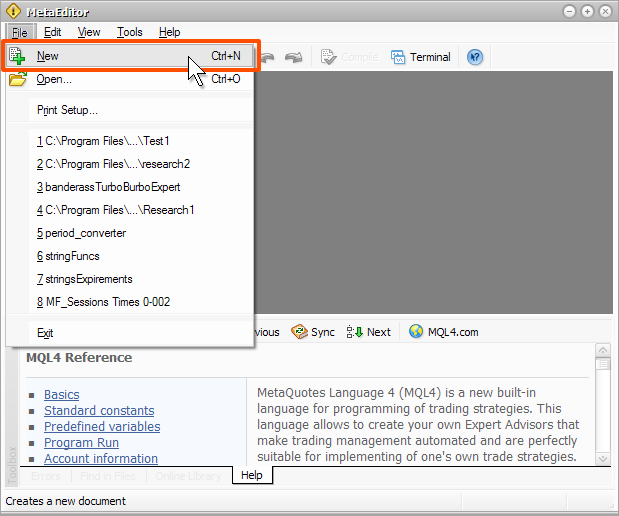In the window that appeared, choose what you are going to create. Choose Script and click Next:In the next window print the name of the script in Name field. Add your name in Author field and e-mail or web address in Link field. Then click OK: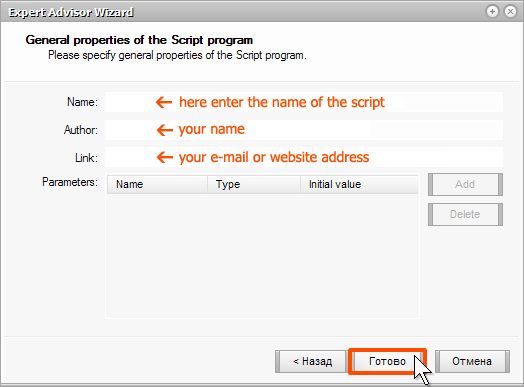After that you will see a new window, with which you will work most of all. It shows the original text: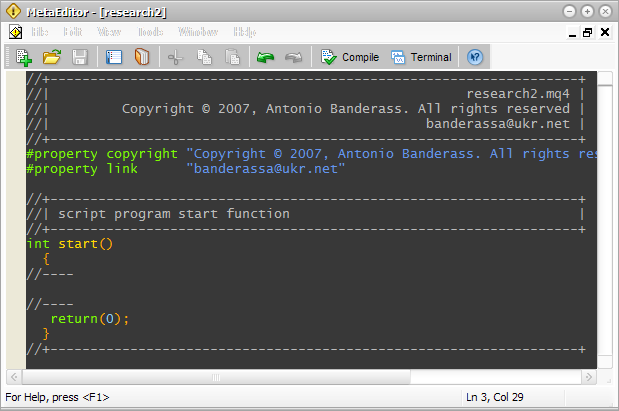Please note, that even an empty not operating script contains a code. Now everything is ready to begin programming. But, unfortunately, you have no idea, how it should be done. Let’s try to improve the situation.

### Source Code, Compiling and Others

You should understand an important matter. What is written in MetaEditor is a source code. I.e. it is a sequence of commands that a terminal will follow one after another downward. But the terminal cannot follow source codes. The source code is understandable for you, but not for MetaTrader. To make the source code understandable for the terminal, it should be “translated” into a proper “language”. To make this, press F5 key in MetaEditor. After that the source code will be compiled into a run file. Compiling is a process of “translating” a source code, written by you and understandable for you, into a special file, which is understandable and performable by MetaTrader. Check it yourself. Create a new script named “Test1” and save, but do not compile. Open the terminal and, using “navigator”, enter “Scripts” folder. You see, there is no script named “Test1”: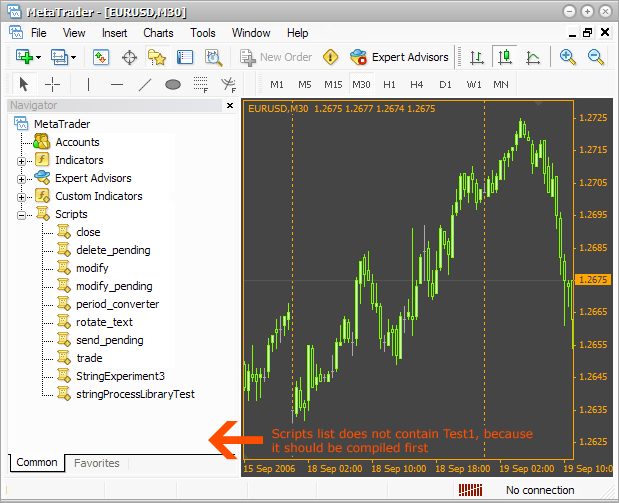Now compile the script (F5 key):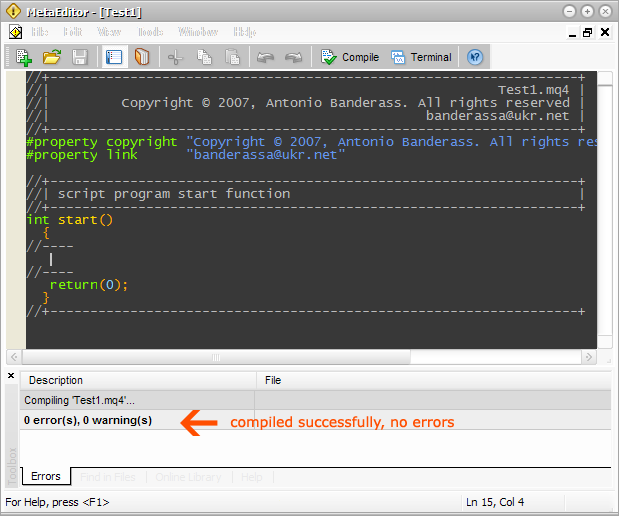Enter the terminal once again. There is a script named “Test1”: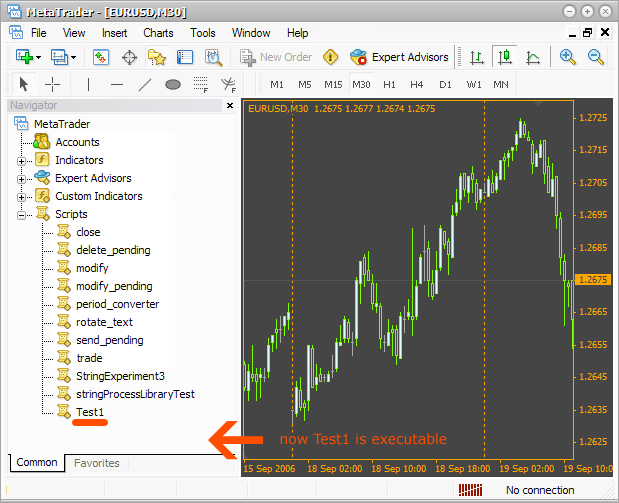Click twice on the script name in the terminal Navigator and it opens. But nothing happens, because the script is empty.

You should already understand, what the process of script writing looks like: you write the source code, compile it, open the script in the terminal, watch the result, change the specific code, compile, watch … and so on until you get the necessary result.

### Where Should Scripts Be Written?

You have already seen, that an empty script contains a specific code. But where should you write the source code, which will work? It should be written between lines int start(){ and return(0);}, as shown at the picture: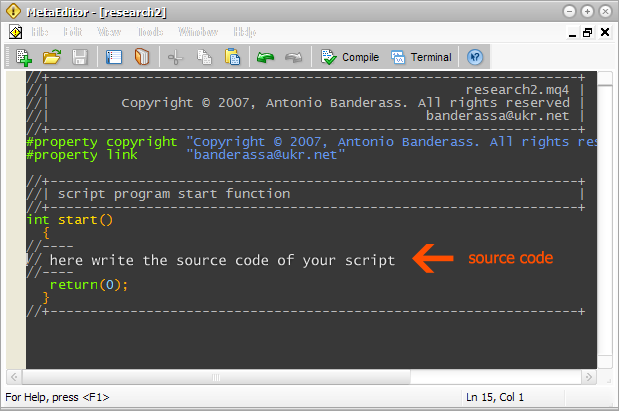Write here everything that I will offer you, and all this will work. Later we will view in details, what these lines mean.

### Variables

What a variable is? Try to answer this question yourself and I will help you. How old are you? How old will you be in five years? Now look: your age is a variable. Your age changes with the course of time, like any other variable. I.e. the first characteristic of a variable is its change with the course of time. Another example: what was your height when you were five? Probably, much less than now. Height is another example of a variable. But there is an important difference. Note, that age is calculated in integers. Height is better assessed in decimals (“floating-point number”). Age: 20 years, 30 years. Height: 1.8 meters, 1.95 meters. This is a very important feature: each variable belongs to a certain type. Let’s see, what other types of variables exist. You can describe in figures many parameters, but how should we describe a text? Special string types are used for this purpose. Such variables types contain only lines. Now let’s see, how to create and describe a variable in MQL4 language. Example:

`int age = 25;`

Here we see an integer type variable (int - integer). Int is a key word of MQL4 language, which shows, that we use a whole number type. Then we wrote “age” – it is the name of a variable, i.e. a word, using which we will reach the meaning, stored in this variable. After that we assigned value 25 to the variable, using symbol “=”. After each instruction “;” should be put. Note, declaration and initialization of any variable come to the variable following form:

[ variable type] [ variable name] = [ variable value];

By the way, it is not necessary to assign a value to a variable (initialize), you could write it so:

`int age;`

One more example:

`double height = 1.95;`

Here we declare a variable named “height”, which stores double type values (floating-point number, decimal), and assign a value 1.95 using “=” operator.

Now let’s see a string variable:

`string name = "Janet";`

string is a variable type, name is a variable name, “Janet” is a variable value. Note, that the value of the string type variable is placed between double quotes (“).

There is another very useful type of a variable – bool. Variables of this type can accept only two values: either true or false. Example:

`bool trend = false;`

Now you should remember some easy things. MQL4 language is a case dependable language, i.e. it is very important, whether you write a code in CAPITALS or small letters. For example if you declare several variables with identical names, but different case, you will get absolutely different variables:

```double HIGHTPRICE;
double hightprice;
double HightPrice;
double hightPrice;```

The above shown code will create four absolutely different variables. Also please note, that all key words of MQL4 language are written in small letters.

Next example:

```DOUBLE hightPrice1;
Double hightPrice2;```

The abovementioned code will not work, because the word “double” will not be accepted as a key word of MQL4 language. One more important moment is that the names of variables can not start with numbers or special signs (*,&,,%,\$). For example:

```double 1price;
double %price;```

One more language element is a comment. If you write “//” before a line, it will whole be commented. It means that during compiling it will be ignored. For example:

`// this is a comment `

Now you can see, that an empty script code includes many comments of an informative character. Comment your code. Sometimes it may help you save a lot of time.

### Working with Variables

Now let’s see, what we can do with variables after we have declared them. Let’s see a simple example:

```double a = 50.0;// declare a value with a floating point and
// assign the value 50 to it
double b = 2.0;
double c;

c = a + b;      // assign to the variable c sum of variables
// a and b. Now the value is equal to 52. Like after
// any other instruction put a semicolon (“;”)

c = a - b;      // diminution, c = 48
c = a*b;        // multiplication, c = 100
c = a / b;      // division, c = 25
c = (a + b)*a;  // place the operations that should be performed
// first inside the brackets. In our case
// first we get the sum of a and b
// after that this sum will be multiplied by a and assigned to c

c = (a + b) / (a - b); // if there are several operations in brackets,
// they will be performed
c = a + b*3.0;  // according to mathematic rules first you will get
// multiplication b and 3, and then the sum```

If you need to perform an operation with a variable and assign a result to it, for example add 5, do it in one of the following ways:

```int a = 5;
a = a + 5;  // add 5
a += 5;     // analogous
a = a*5;
a *= 5;     // multiply a by 5 and assign to it
a /= 5;     // divide and assign```

If you want to add or subtract 1, use the following method:

```int a = 5;
a++;  // add1, it is called increment
а--;  // subtract 1, it is decrement```

It is all ok, but working with such scripts you can only guess, whether everything goes correctly, because nothing happens on the screen.

That is why it is convenient to show the results. For this purpose there is an integrated function MessageBox().

### MessageBox()

A function is a set of instructions, which accepts the parameters and depending on them shows the result. In our case MessageBox() function accepts two parameters: first – message text, second – title text. Here is an example:

`MessageBox("Hello, World! There is some text.","caption");`

To perform a function, first write its name. Do not forget about the case! Then open the brackets and write parameters using commas. In our case parameters are of a string type. As we remember, all lines are written inside quotes (“). Like in any instruction, put a semicolon at the end. To understand properly let’s see the picture. It shows how the code and results are bound: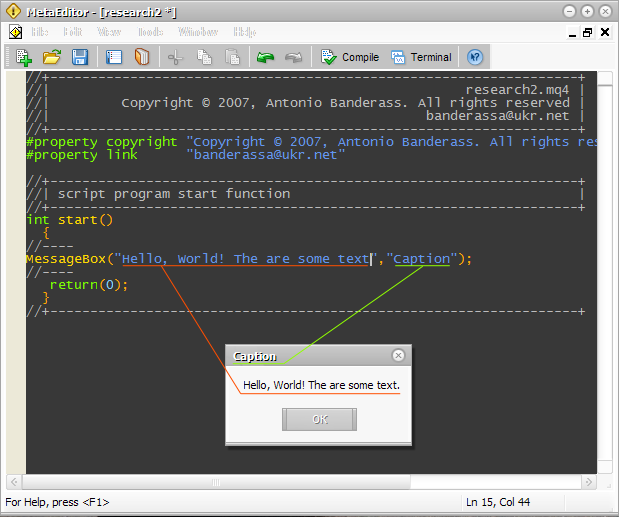Of course, it is all ok. But how can we show variables of other types? Easily – make a mental note:

```int a = 50;
int b = 100;
MessageBox("It is very simple. a+b=" + (a + b), "a+b=?") ```

As a result we get: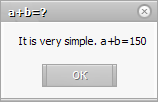As you have already guessed, MQL4 is designed so, that when we try to add another number type to a line, it automatically transfers numbers into lines and combines them. It is a really wonderful property! You can do such operations with string variables also:

```int a = 50;
int b = 100;
string str1 = "a + b =";
str1 += a + b; // now str1 = "a + b = 150"
// now use the variable str1 as
// a first parameter
MessageBox(str1, "a + b = ?");```

Now you know how to draw different data using MessageBox() function. But what is the interest of showing the results of simple mathematic operations? We need MQL4 not simply to find sums and multiplications, don’t we?

### Arrays

Do not be afraid. It is very easy. Look. Suppose you want to remember five prices. What should we do? Well, we can do this:

```double price1 = 1.2341;
double price2 = 1.2321;
double price3 = 1.2361;
double price4 = 1.2411;
double price5 = 1.2301;```

We get five variables, which have one data type and describe one parameter – price. We can go another way, using an array. An array is a set of variables, which differ in indexes, but have one name. This is how we declare an array from five elements:

`double price;`

Common form:

(array type) (array name) [number of elements];

In our case: array type – double, name – price, number of elements – 5. Let’s see how we can refer to the array elements:

```double price;   // declare an array of 5 elements

price = 1.2341; // refer to the first element of the array and
// assign a price to it. Note
// that the index of the first element starts with 0
// It is an important feature,
// you should get used to it.

price = 1.2321;  // refer to the second element
price = 1.2361;  // and so on
price = 1.2411;
price = 1.2301;
```

We can perform any operations with the array elements, like with common variables. Actually, elements of the array are common variables:

```double price;
price = 1.2234;
price = 1.2421;
MessageBox("Middle price is " + (price +
price) / 2.0,"middle price");
```

When declaring an array we can assign initial values to all elements:

`double price = {1.2234, 1.2421};`

We simply enumerate the initial values of elements comma separated in braces. In such a case you may let a compilator automatically put the number of elements, instead of writing it yourself:

`double price[] = {1.2234, 1.2421};`

Undoubtedly all this is ok, but… Unfortunately it is useless. We should somehow get to real data! For example, current prices, time, amount of free money and so on.

### Integrated or Built-in Arrays and Variables

Of course we cannot do without real data. To get access to them, we should simply refer to a corresponding built-in array. There are several such arrays, for example:

```High;   // refer to the last maximal price,
// that the bar has achieved in the current timeframe
// and current currency pair. Currency pair and
// the timeframe depend on the chart, on which you
// have started a script. It is very important to remember!

Low;    // minimal price of the last bar
// in the current chart.

Volume; // value of the last bar in the current chart.```

To understand properly in-built arrays and indexes, look at this: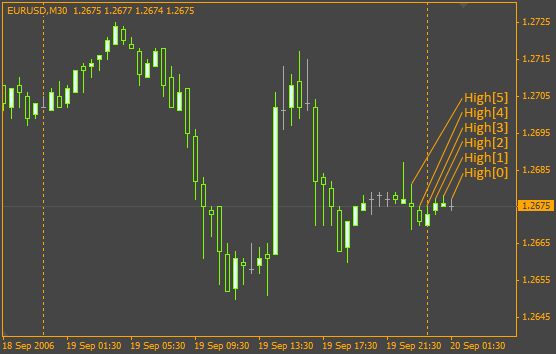You see, the last bar index (number) is 0, next to last is 1 and so on.
There are also built-in common variables. For example Bars shows the number of bars in the current chart. It is a common variable, but it was declared before you and outside your script. This variable exists always, like other built-in arrays and variables.

### Cycles

Suppose you decided to calculate a mean value of maximal prices of all bars in the chart. For this purpose you alternatively add each element to a variable, for example so:

```double AveragePrice = 0.0;
AveragePrice += High;
AveragePrice += High;
AveragePrice += High;
AveragePrice += High;
AveragePrice += High;   // ... and so on
AveragePrice /= Bars;```

I can tell you only this: it may work, but it is absurd. For such purposes there are cycles. Note, that all operations are absolutely similar, only the index changes from 0 to variable Bars-1 value. It would have been much more convenient somehow to determine a counter and using it refer to the array elements. We can solve this task using cycles:

```double AveragePrice = 0.0;
for(int a = 0; a < Bars; a++)
{
AveragePrice += High[a];
}```

Let’s see each line:

```double AveragePrice = 0.0; // everything is clear
// this is a cycle.
or(int a = 0; a < Bars; a++)```

You see, the cycle starts with a key word for. (There are other types of cycles, for example while, but we will not learn them now). Then in quotes point semicolon separated counter, cycle operation conditions, counter increase operation. In a general case it can be presented like this:

```for(declaration of a counter; the cycle operation conditions;
counter changes)
{
// the source code, that will be repeated is in braces,
}```

Let’s view each stage of cycle declaration more closely.

Count declaration: int type is used for the counter. The name of the variable-counter does not matter. You should initialize the primary value, for example by 0.

Counter operation conditions: it is all easy. Here you determine a condition, and if it is true, the cycle goes on. Otherwise the cycle is ended. For example in out case:

`a < Bars`

It is clear, that the cycle will go on while the variable-counter is less than variable Bars. Suppose, variable Bars=10, then at each move over the cycle, the variable will increase by 1 until it reaches 10. After that the cycle stops.

Counter change: if we do not change the counter (in our case increase it)? The cycle will never end, because the condition will never be fulfilled. For better understanding of the cycle meaning, I have written a code that will perform a cycle with describing comments:

```// the cycle:
// double AveragePrice=0.0;
//
// for(int a=0;a>
// {
//   AveragePrice+=High[a];
// }
//
// will be performed in this way:
//

double AveragePrice=0.0;

int a=0;

AveragePrice+=High[a];
a++;                    // now a=1, suppose Bars=3.
// then the cycle goes on, because Bars > a

AveragePrice+=High[a];
a++;                   // a=2

AveragePrice+=High[a];
а++;                   // a=3

// the conditions is not fulfilled any more, so the cycle
// stops, because a=3 and Bars=3```

Now you should understand how the cycle works. But you should know some more details.

Cycle operation conditions can be different. For example like this:

```a>10    // the cycle works while a>10
a!=10   // the cycle works while a is not equal to 10
a==20   // while a is not equal to 20
a>=2    // while a is more or equal to 2
a<=30   // while a is less or equal to 30
```

The counter can be changed in a different way. For example, you do not have to increase it each time by 1. You can do like this:

```a--     // the counter will each time decrease by 1
a += 2  // the counter will each time increase by 2
```

Beside, you can place the counter change inside the cycle body. For example so:

```for(int a=0; a<Bars;)
{
AveragePrice+=High[a];

a++; // the counter changes inside the cycle body
}
>```

Also it is not necessary to declare the variable-counter in the cycle. You can do it another way:

```int a = 0;

for(;a < Bars;)
{
AveragePrice += High[a];

a++; // the counter changes inside the cycle body
}```

If the cycle body contains only one operator, for example like this:

```for(int a = 0; a < Bars; a++)
{
AveragePrice += High[a];
}```

then it is not necessary to put braces:

```for(int a = 0; a < Bars; a++)
AveragePrice += High[a];```

It is all about cycles by now. There are other cycle types, but we will discuss them at the next lesson. Now you should get, when to use the cycles and remember the syntax. Try to write several cycles, that will show the counter values through MessageBox() function. Try to write a discontinuous cycle and see what will happen after start.

### Conditions

There is one more important thing that you will always use – conditions. Our life contains a lot of conditions and action that follow these conditions. Very often we think by conditional clause. For example: “If I have enough time, I will read this book. If not, then it’s better to read a magazine”. You can generate hundreds of such conditions and actions. But how can we write them in MQL4? Here is an example:

```// of course the conditionsn should be written in MQL4
if( I have enough time )
{
// here we place any actions, directions in MQL4
}
// if the first condition is not fulfilled,
// the second is fulfilled
else
{
}
```

Now you should understand the syntax of conditions. Let’s view conditions, fully written in MQL4 language:

```int a = 10;
int b = 0;

if(a > 10 )
{
b = 1;
}
else
{
b = 2;
}

MessageBox("b=" + b,".");
```

Everything is quite easy. What is the value of b after the completion? Of course b=2, because the condition a > 10 is not fulfilled. It is easy. By the way, it is not necessary to use the key word else:

```int a = 10;
int b = 0;

if(a > 10)
{
b = 1;
}```

In this case if a condition is not fulfilled, the code block that follows the condition inside braces is omitted. In our case after fulfilling b = 0. Also pay attention on the way, the conditions are built. We know that different types of variables can acquire different values, for example:

• int - integers (1, 60, 772);
• double – with a floating point (1.0021, 0.221);
• string – only lines ("Word", "Some text.");
• bool – only true or false (true, false).

So, the conclusion is: in conditions compare variables only with the values, they can accept, for example:

```int Integer=10;
double Double=1.0;
string String="JustWord";
bool Bool=true;

if(Integer<10) // the condition is correct
{
MessageBox("It works!","Really!");
}

if(Double!=1.0) // the condition is correct
{
MessageBox("works!","Simple!");
}

if(String==10) // this is nonsense!!
// we found the variable
//String with string type, but 10 is int
{
MessageBox("Wrong type","u can't c this");
}

if(String!="Word") // ok
{{
// ...
}

if(Bool==true) // correct
{
// ...
}
```

Note, that we make comparisons using condition operators (==, !=, >, <, >=, <=). For string and bool types use only == and != comparisons.

Now let’s get acquainted with the meaning of inclusions. Yes, you can use conditions in cycles and cycles in conditions; you can use conditions in other conditions and so on. For example:

```int a=0;
double b=0.0;
bool e;

if(a==0)
{
for(int c=0;c<Bars;c++)
{
b+=High[c];
}

if(b>500.0)
{
e=true;
}
else
{
e=false;
}
}```

The next example shows another way of using conditions:

```int a=0;
int result;

if(a==0)
{
result=1;
}
else if(a==1)
{
result=2;
}
else if(a==2)
{
result=3;
}
else
{
result=4;
}```

If in case of one condition default you have another condition, write it after the key word “else”, like in the code above. This will also work. The number of other conditions is unlimited. If actions of condition fulfillment fit one operation, you may omit braces like in cycles:

```if(a==1)
{
b=2;
}

// or this way:

if(a==1)
b=2;```

### Complex Conditions

Very often one condition is not enough. You need to compare many parameters; here you have to do with complex conditions. For example, if I have enough time and patience, I will learn MQL4 language. We can write it as a code:

```if((enough time) && (enough oatience))
{
I will learn MQL4 language;
}
```

It means you should first divide complex conditions into simple conditions, put them into brackets, and put && (logical AND) or || (logical OR) between them. If both conditions should be true, put && (AND). If only one conditions should be true, put || (OR). Here is an example:

```nt a=10;
int b=20;

if((a>5) && (b<50))
MessageBox("Its works!","Yes");```

Beside you can enclose and combine as much conditions, as you need:

```int a=10;
int b=20;

if ( ((a>5) || (a<15)) && ( b==20))
MessageBox("It works, too!","Yes");```

### All Together

Using together complex and simple conditions, cycles you may write a very complicated code. Almost every mechanism can be described using these plain structures of MQL4 language. If you understand, how these simple things are written and operate, you will understand a half of MQL4 or any other programming language! It is really very easy! All you need is practice. Try to write as much scripts as you can, in order to remember the syntax and get the practice. Besides, view the samples in the attached file examples.mq4 and try to understand them.

### Other Built-in Variables and Arrays

You already know such arrays as High[], Low[], Volume[] and a variable Bars. Here are some more useful variables:

```double Open[]  // the array of prices of the current
// chart opening bars
double Close[] // the array of prices of the current
// chart closing bars

double Bid  // the last known buying price in
// the current currency pair
double Ask  // the last known selling price in
// the current currency pair```

### Conclusion

Attached files |
examples.mq4 (3.11 KB)
Last comments | Go to discussion (12)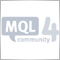| 12 May 2010 at 06:35
```// of course the conditionsn should be written in MQL4
if( I have enough time )
{
// here we place any actions, directions in MQL4
}
// if the first condition is not fulfilled,
// the second is fulfilled
else
{
}
```

Now you should understand the syntax of conditions. Let’s view conditions, fully written in MQL4 language:

Your tutorial is well written but fails where almost all the mql4 documentation I have read fails, on the syntax description. At no point did you describe or mention why braces should be used, what they are for and what will happen if they are put in the wrong place, the same goes for parenthisis, this is probably on of the biggest problem people new to programming face when they try to learn mql4 and it seems none of the tutorial writers have any comprehension of this, you did go some way to explaining the use of parenthesis but even that was a hazy kind of by the way explanation, if you really want to help newbies learn mql4, start by writing a tutorial properly explaining the syntax, especially the use of braces, parenthesis and semi colons.| 24 Mar 2013 at 22:52

Hello everyone.

Excuse my English.

I have seven years doing trading and only half month learning MQL4. I have some questions.

In this chart suppose:

Highs
Price
High
1.2676
High1.2677
High1.2676
High1.2675
High1.2675
High 1.2680

About the example 2 in examples.mq4:

```double maxAverage=0.0;
double minAverage=0.0;

for(a=0;a<Bars;a++)
{
maxAverage+=High[a];
minAverage+=Low[a];
}

maxAverage/=Bars+1;
minAverage/=Bars+1;

MessageBox("maxAverage="+maxAverage+" minAverage="+minAverage,"max and min averages");```

Questions:

1) In the line

`for(a=0;a<Bars;a++)`

I understand that Bars = 6 and "a" takes the values, ¿is this correct?

 a = 0 a = 0+1 a = 1+1 a = 2+1 a = 3+1 a = 4+1

2) Is maxAverage+=High[a] calculated as follows?

a =
High
maxAverage = maxAverage + High[a]
0
1.2676
1.2676 = 0 + 1.2676
1
1.26772.5353 = 1.2676 + 1.2677
2
1.26763.8029 = 2.5353 + 1.2676
3
1.2675
5.0704 = 3.8029 + 1.2675
4
1.2675
6.3379 = 5.0704 + 1.2675
5
1.26807.6059 = 6.3379 + 1.2680

3) How to find the average maximal price with this line?

`maxAverage/=Bars+1;`

maxAverage = maxAverage /(Bars+1)

maxAverage = 7.6059 / (6+1) ? => wrong answer.

or

maxAverage = (7.6059 / 6) + 1 => wrong answer again.| 2 Apr 2017 at 00:40

Hello there,

I'm pretty new and I somehow don't seem to extract all knowledge there is from your text

But you don't walk me through as you advertise. I don't seem to get it right.

For example If I'd like to do something like this:

void OnStart();
{
double AveragePrice = 0.0;
int a = 0;
for(int a = 0; a < Bars; a++)
AveragePrice += High[a];
MessageBox("AveragePrice= " + AveragePrice, "Average Price");
return(0);
}

So I want the script to give the average high price to me in a MessageBox, that should not be too difficult, but the problem keeps finding mistakes if I compile.

It says there is something wrong with the opening brace "{". What seems to be the problem here? It's really frustrating.

And maybe you can write everything down to a "t" so that real newbies can see where the mistakes are, that would be terrific.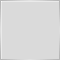| 2 Apr 2017 at 01:08

You shouldn't have a semicolon after OnStart()

```void OnStart();
{
double AveragePrice = 0.0;
int a = 0;
for(int a = 0; a < Bars; a++)
AveragePrice += High[a];
MessageBox("AveragePrice= " + AveragePrice, "Average Price");
return(0);
}```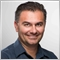| 7 Sep 2017 at 17:12

good job!!

I learn a lot, thank you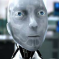How to Develop a Reliable and Safe Trade Robot in MQL 4

The article deals with the most common errors that occur in developing and using of an Expert Advisor. An exemplary safe automated trading system is described, as well.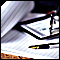Modelling Requotes in Tester and Expert Advisor Stability Analysis

Requote is a scourge for many Expert Advisors, especially for those that have rather sensitive conditions of entering/exiting a trade. In the article, a way to check up the EA for the requotes stability is offered.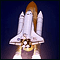Running MetaTrader 4 Client Terminal on Linux-Desktop

Description of a step-by-step Linux-desktop setup using a non-emulator wine for running MetaTrader 4 Client Terminal on it.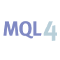MQL4 Language for Newbies. Difficult Questions in Simple Phrases.

This is the second article from the series "MQL4 Language for Newbies". Now we will examine more complex and advanced constructions of the language, learn new options and see, how they can be applied in everyday practice. You will get acquainted with a new cycle type 'while', new condition type 'switch', operators 'break' and 'continue'. Besides you will learn to write your own functions and work with multidimensional arrays. And for a dessert I have prepared an explanation about a preprocessor.